# Java code to arrange the letters of a word in alphabetical order

Java program to arrange the letters of a word in alphabetical order.

```import java.io.*;
class AlphabeticalOrder
{
static String n;
static int l;
public static void main(String args[]) throws IOException
{
System.out.print("Enter a word : ");
l = n.length();
alphabetical();
}
public static void alphabetical()
{
char b[] = new char[l];
for(int i=0;i<l;i++)
b[i] = n.charAt(i);
char t;
for(int j=0;j<l-1;j++)
{
for(int k=0;k<l-1-j;k++)
{
if(b[k]>b[k+1])
{
t=b[k];
b[k]=b[k+1];
b[k+1]=t;
}
}
}
System.out.println("\nOriginal word : " +n);
System.out.print("Sorted word : ");
for(int m=0;m<l;m++)
System.out.print(b[m]);
System.out.print("\n");
}
}
```

ALGORITHM:-

1. Start

2. Accept a word from the user.

3. Convert each letter of the word to its corresponding ASCII value.

4. Compare each letter of the word by its next letter and swap them if the first letter has a greater ASCII value than the next one.

5. Repeat the above procedure until the entire word is sorted.

6. Print the new word.

7. End

OUTPUT:-

Enter a word : computer

Original word : computer

Sorted word : cemoprtu

Related Programs:-

Display the solution of Towers of Hanoi using recursion

Convert a number into words

Reverse a word or sentence ending with '.' using recursion

Convert Decimal to Octal

Convert Decimal to Binary

1.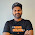2.Anonymous5:44 pm

Share java code for print string without specific letter.
Ex: Input value-- selva@gmail.com
Output value-- selvagmailcom (Remove @&.)

3.////can i write like this......? ///

class Abcd
{
public static void main(String args[])
{
int i=0,j=0;
char a1[]={'a','b','c','d','e','f','g','h','i','j','k','l','m','n','o','p','q','r','s','t','u','v','w','x','y','z'};
char b1[]={'r','a','k','e','s','h'};
for(i=0;i<=25;i++)
for(j=0;j<=5;j++)
{
if(a1[i]==b1[j])
System.out.println(a1[i]);
}
}
}

1.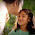This comment has been removed by the author.

2.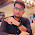good bro...

4.very useful

5.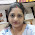Thank You

1.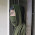Welcome

6.can anyone please give me the code for:
If i am entering any word which is not in arranged manner then after clicking enter it should give the rearrange this with meaningfull word at console page.
Word string length may consist 0-infinite length of letters

7.Faltu hai

8.9.10.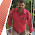arrange the string given order.
input : ( pro@gr98am)
output : (program@98)

11.Write A Program for this:
Input-This is a zoo
Output-sihT si a ooz

12.public class Alphabets
{
public void disp(String str)
{
int i,j,p;
char chr;
p=str.length();
for(i=65;i<=90;i++)
{
for(j=0;j<p;j++)
{
chr=str.charAt(j);
if(chr==(char)i||chr==(char)(i+32))
System.out.println(chr);
}
}
}
}
//a better program

13.14.Excellent site. Lots of useful info here. I am sending it to several friends ans also sharing in delicious. And certainly, thanks for your effort!engaging content

15.Learning Code Examples learn samples about java

16.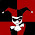Thanks for sharing all of this wealthy information. It’s not easy to get such quality information online nowadays. I also want to share with you some good website - https://essay4me.org/. Great team, excellent writers and very professional support.